GATE  >  Test: General Aptitude - 1

# Test: General Aptitude - 1

Test Description

## 20 Questions MCQ Test GATE Physics Mock Test Series | Test: General Aptitude - 1

Test: General Aptitude - 1 for GATE 2022 is part of GATE Physics Mock Test Series preparation. The Test: General Aptitude - 1 questions and answers have been prepared according to the GATE exam syllabus.The Test: General Aptitude - 1 MCQs are made for GATE 2022 Exam. Find important definitions, questions, notes, meanings, examples, exercises, MCQs and online tests for Test: General Aptitude - 1 below.
Solutions of Test: General Aptitude - 1 questions in English are available as part of our GATE Physics Mock Test Series for GATE & Test: General Aptitude - 1 solutions in Hindi for GATE Physics Mock Test Series course. Download more important topics, notes, lectures and mock test series for GATE Exam by signing up for free. Attempt Test: General Aptitude - 1 | 20 questions in 40 minutes | Mock test for GATE preparation | Free important questions MCQ to study GATE Physics Mock Test Series for GATE Exam | Download free PDF with solutions
 1 Crore+ students have signed up on EduRev. Have you?
Test: General Aptitude - 1 - Question 1

### From a container of 8 litre pure syrup 1 litre is taken out and replaced by water of equal quantity. If the same repeated again then what is the present ratio of syrup to water.

Detailed Solution for Test: General Aptitude - 1 - Question 1

Each time we take 1 litre from 8 litre, 7 litre is left. So, the original: remaining of syrup is
8:7
So, each tune we take out there would be 7/8th part left of the syrup.
8 lit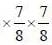(as two tunes done)
=49/8 litre syrup left
Therefore, water = total - remaining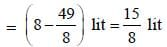Therefore, syrup left: water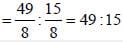*Answer can only contain numeric values
Test: General Aptitude - 1 - Question 2

### In a family a father has three daughters and each daughter has a brother. The number of known male members in the family is___________

Detailed Solution for Test: General Aptitude - 1 - Question 2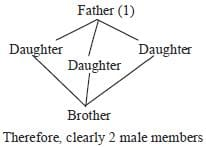Test: General Aptitude - 1 - Question 3

### (i) The speed of your bike should not (ii) exceed more than 70 km/hr (iii) as you are driving alone (iv) No error In the above sentene if there is any mistake, mark the corresponding part. e.g. if there is a mistake in part (i) please mark (i) as your answer and similarly for other options. If no mistake is there mark ‘no error' (iv) as your answer

Detailed Solution for Test: General Aptitude - 1 - Question 3

Exceed is not followed by “more than'"

Test: General Aptitude - 1 - Question 4

A cricketer’s total run before the end of a senes is 750. After that he plays two more matches in the same series in which he scores 6 runs and his battmg average decreases by 2 runs. What is his batting average for the series

Detailed Solution for Test: General Aptitude - 1 - Question 4

Let he has played x matches
Therefore, average 750/x runs/match.
After scoring 6 mroe runs, his total is (750 + 6) = 756 runs To score 6 more runs he has played 2 more matches Therefore, total matches played = (x + 2)
Therefore, now average runs/match = 756/x+2
As per condition,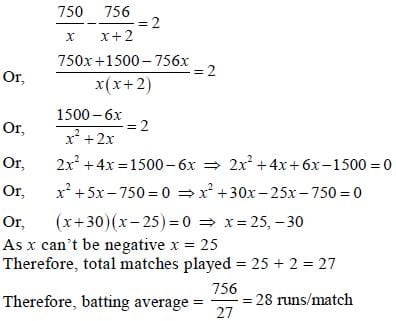Test: General Aptitude - 1 - Question 5

Rain can do a work in 28 days while the same work can be done by Shyam m 35 days. They started Unseen the work together but after some days, Ram left the work and Shyam completed the remaining work in 17 days. After how many days Ram left work.

Detailed Solution for Test: General Aptitude - 1 - Question 5

Let total work = x
Therefore, one daywork of Ram = x/28
Therefore, one day work of Shyam= x/35
In 17 days work of Shyam =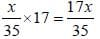Remaining work =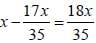Now, in 1 day Ram and Shyam does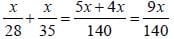∴ 9x/140 = work done in 1 day
1 work done in 140 / 9x days
18x / 35 work done in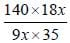= 8 days
Therefore, after working 8 days Rani left

Test: General Aptitude - 1 - Question 6

Ramesh standing on a platform observes tliat a train crosses him in 8 sec. Another train of same length from opposite direction crosses him in 6 secs. Running in opposite direction how much tune it would take to cross each other for the trains.

Detailed Solution for Test: General Aptitude - 1 - Question 6

Total distance to be covered to cross each other is the total length of both the trains. If length of both the trains are x. Then total distance to be covered = 2x First train in 8 secs goes x
First train in 1 secs goes x/8
Second train in 6 secs goes x
Second train in 1 secs goes x/6
Therefore, in 1 sec goes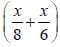(as in opposite direction)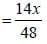Now, 14x / 48  goes m 1 sec
Therefore, 2x goes in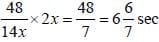*Answer can only contain numeric values
Test: General Aptitude - 1 - Question 7

Anil rows 30 km downstream in 10 hours. Same time is also taken to row 18 km upstream. Then, the velocity of water is __________ km/hr.

Detailed Solution for Test: General Aptitude - 1 - Question 7

Down stream speed = 30/10 = 3km/hr = U+V
Up stream speed = 18/10 = 1.8 km/hr = U-V
Velocity of water =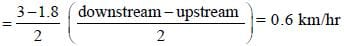Test: General Aptitude - 1 - Question 8

If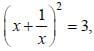then value of x18 + x12 + x6 +1 = ?

Detailed Solution for Test: General Aptitude - 1 - Question 8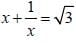∴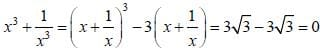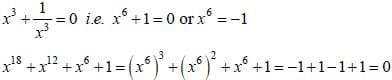Test: General Aptitude - 1 - Question 9

A Rubber ball is thrown from a height of 600 m and each time it bounces back 2/3 of the last bounce. Unseen What is the total distance covered by the ball before it stops

Detailed Solution for Test: General Aptitude - 1 - Question 9

Falling from 600 and again going up by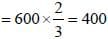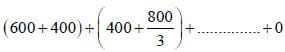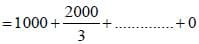Each next term is being multiplied by 2/3
a= 1000, r = 2/3 i.e. G.P. series
Therefore, sum =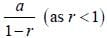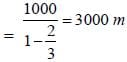Test: General Aptitude - 1 - Question 10

What is the minimum value 0f 4 secθ + 9 cosec2 θ = ?

Detailed Solution for Test: General Aptitude - 1 - Question 10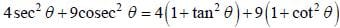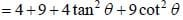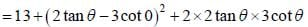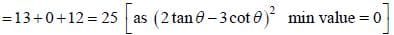*Answer can only contain numeric values
Test: General Aptitude - 1 - Question 11

Two poles of equal height are standing opposite to each other on the two sides of a 100 wide road. From a point on the road the angle of 30° and 60° are formed with the top of the two towers. The height of the first pole in metre is ________________ (upto two decmial places)

Detailed Solution for Test: General Aptitude - 1 - Question 11

Let us assume that we are standing at a point E somewhere on the road from where we are spectating.
Now, two poles are AB and CD; equal height i.e. AB = CD
From,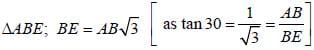And similarly from ΔCDE,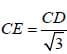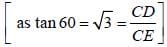∴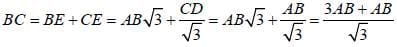∴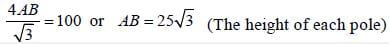Test: General Aptitude - 1 - Question 12

If all the circles have a radius of 10 cm, have a radius of 10 cm, then what is the area of the extrapped region between circles.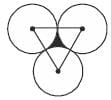Detailed Solution for Test: General Aptitude - 1 - Question 12

Area in between = area of the triangles - are covered by circles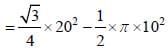(As each side of the equilateral triangle is 20 m and as angle of total 180° is covered by circles, i.e. half of 360°)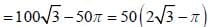Test: General Aptitude - 1 - Question 13

A metallic right circular cone of height 12 cm and base of 7 cm radius is melted and recasted to form a cuboid having breadth and height of 11cm and 2cm. What is the length of cuboid.

Detailed Solution for Test: General Aptitude - 1 - Question 13

Volume of cone = volume of cuboid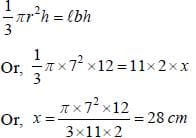Test: General Aptitude - 1 - Question 14

Count total number of triangles in the given figure.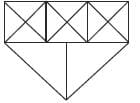Detailed Solution for Test: General Aptitude - 1 - Question 14

Total triangles, in the upper part = 28
And in lower part is 3
therefore, total triangle is 28 + 3 = 31

Test: General Aptitude - 1 - Question 15

If in a certain code ‘SCHOOLS’ is written as ‘IDTPTMP’, then what will be the code for ‘SUCCESS’.

Detailed Solution for Test: General Aptitude - 1 - Question 15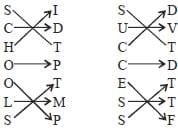Test: General Aptitude - 1 - Question 16

In how many ways a football team can be formed out of 15 players where the captain is fixed and always in the team.

Detailed Solution for Test: General Aptitude - 1 - Question 16

One player is always fixed, so we have to choose 10 players, out of 14,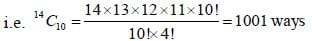Test: General Aptitude - 1 - Question 17

Some crows are cows
All cows are cats; All cats are rats; Some rats are bats Conclusions,
(1) Some bats are cats
(2) Some crows are rats
(3) Some cows are bats

Detailed Solution for Test: General Aptitude - 1 - Question 17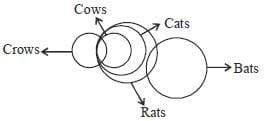So, only intersection between crows and Rats;
Therefore, only 2 Follows.

*Answer can only contain numeric values
Test: General Aptitude - 1 - Question 18

Find the missing number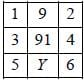Based on the pattern followed above the number of inplace of Y is____________

Detailed Solution for Test: General Aptitude - 1 - Question 18

1+ 23 = 9
33 + 43 = 91
53 + 63 = 341

Test: General Aptitude - 1 - Question 19

What is the total area covered by |x|+|y|=8

Detailed Solution for Test: General Aptitude - 1 - Question 19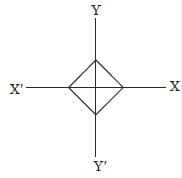In each quadrant area is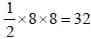square unit
Therefore, in all four quadrants area is 32 x 4 = 128 square unit

Test: General Aptitude - 1 - Question 20

Find out the correct spelling

## GATE Physics Mock Test Series

1 docs|34 tests
 Use Code STAYHOME200 and get INR 200 additional OFF Use Coupon Code
Information about Test: General Aptitude - 1 Page
In this test you can find the Exam questions for Test: General Aptitude - 1 solved & explained in the simplest way possible. Besides giving Questions and answers for Test: General Aptitude - 1, EduRev gives you an ample number of Online tests for practice

1 docs|34 tests